# Atomic StructurePage 1

#### WATCH ALL SLIDES

Slide 1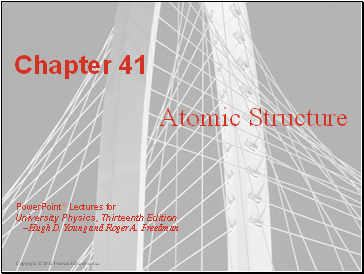Chapter 41

Atomic Structure

Slide 2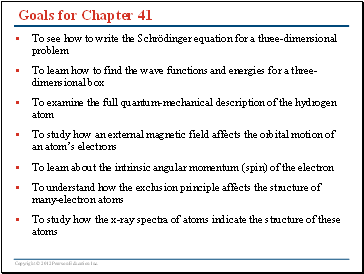Goals for Chapter 41

To see how to write the Schrödinger equation for a three-dimensional problem

To learn how to find the wave functions and energies for a three-dimensional box

To examine the full quantum-mechanical description of the hydrogen atom

To study how an external magnetic field affects the orbital motion of an atom’s electrons

To learn about the intrinsic angular momentum (spin) of the electron

To understand how the exclusion principle affects the structure of many-electron atoms

To study how the x-ray spectra of atoms indicate the structure of these atoms

Slide 3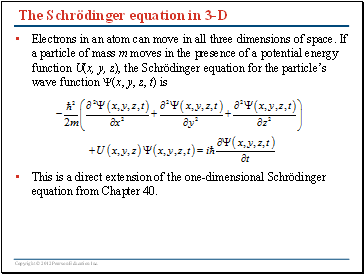## The Schrödinger equation in 3-D

Electrons in an atom can move in all three dimensions of space. If a particle of mass m moves in the presence of a potential energy function U(x, y, z), the Schrödinger equation for the particle’s wave function (x, y, z, t) is

This is a direct extension of the one-dimensional Schrödinger equation from Chapter 40.

Slide 4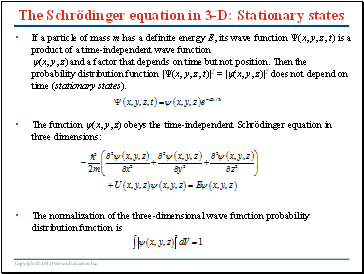The Schrödinger equation in 3-D: Stationary states

If a particle of mass m has a definite energy E, its wave function (x, y, z, t) is a product of a time-independent wave function (x, y, z) and a factor that depends on time but not position. Then the probability distribution function |(x, y, z, t)|2 = |(x, y, z)|2 does not depend on time (stationary states).

The function (x, y, z) obeys the time-independent Schrödinger equation in three dimensions:

The normalization of the three-dimensional wave function probability distribution function is

Slide 5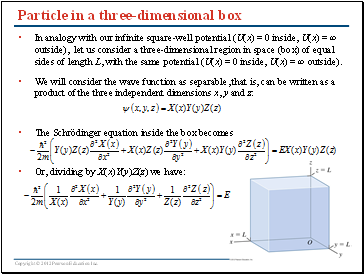## Particle in a three-dimensional box

In analogy with our infinite square-well potential (U(x) = 0 inside, U(x) = ∞ outside), let us consider a three-dimensional region in space (box) of equal sides of length L, with the same potential (U(x) = 0 inside, U(x) = ∞ outside).

We will consider the wave function as separable, that is, can be written as a product of the three independent dimensions x, y and z:

The Schrödinger equation inside the box becomes

Or, dividing by X(x)Y(y)Z(z) we have:

Slide 6Particle in a three-dimensional box

This says that the total energy is contributed to by three terms on the left, each depending separately on x, y and z. Let us write E = Ex + Ey + Ez. Then this equation can be separated into three equations:

Go to page:
1  2  3  4  5  6